Energy Efficiency Analysis

## Background

This project performs energy efficency analysis using 12 different building shapes simulated in Ecotect. The buildings differ with respect to the glazing area, the glazing area distribution, and the orientation, amongst other parameters. Various settings are simulated as functions of the afore-mentioned characteristics.

### Dataset

The dataset comprises 768 samples and 8 features, aiming to predict two real valued responses. The dataset can be downloaded here: https://archive.ics.uci.edu/ml/datasets/Energy+efficiency.

Let load and glance through the data

## 'data.frame':    768 obs. of  10 variables:
##  $Relative.Compactness : num 0.98 0.98 0.98 0.98 0.9 0.9 0.9 0.9 0.86 0.86 ... ##$ Surface.Area             : num  514 514 514 514 564 ...
##  $Wall.Area : num 294 294 294 294 318 ... ##$ Roof.Area                : num  110 110 110 110 122 ...
##  $Overall.Height : num 7 7 7 7 7 7 7 7 7 7 ... ##$ Orientation              : int  2 3 4 5 2 3 4 5 2 3 ...
##  $Glazing.Area : num 0 0 0 0 0 0 0 0 0 0 ... ##$ Glazing.Area.Distribution: int  0 0 0 0 0 0 0 0 0 0 ...
##  $Heating.Load : num 15.6 15.6 15.6 15.6 20.8 ... ##$ Cooling.Load             : num  21.3 21.3 21.3 21.3 28.3 ...

## Cleaning the dataset

Notice that the variable names have a dot in between. Let’s, remove dots from column names.

Lets analyse the response variables in a little detail

library(ggplot2)
## Warning: package 'ggplot2' was built under R version 3.2.2
ggplot(eeframe, aes(x=HeatingLoad, y=CoolingLoad))+
geom_point(color="blue")+
ggtitle("Fig1. Heatling Load vs. Cooling Load")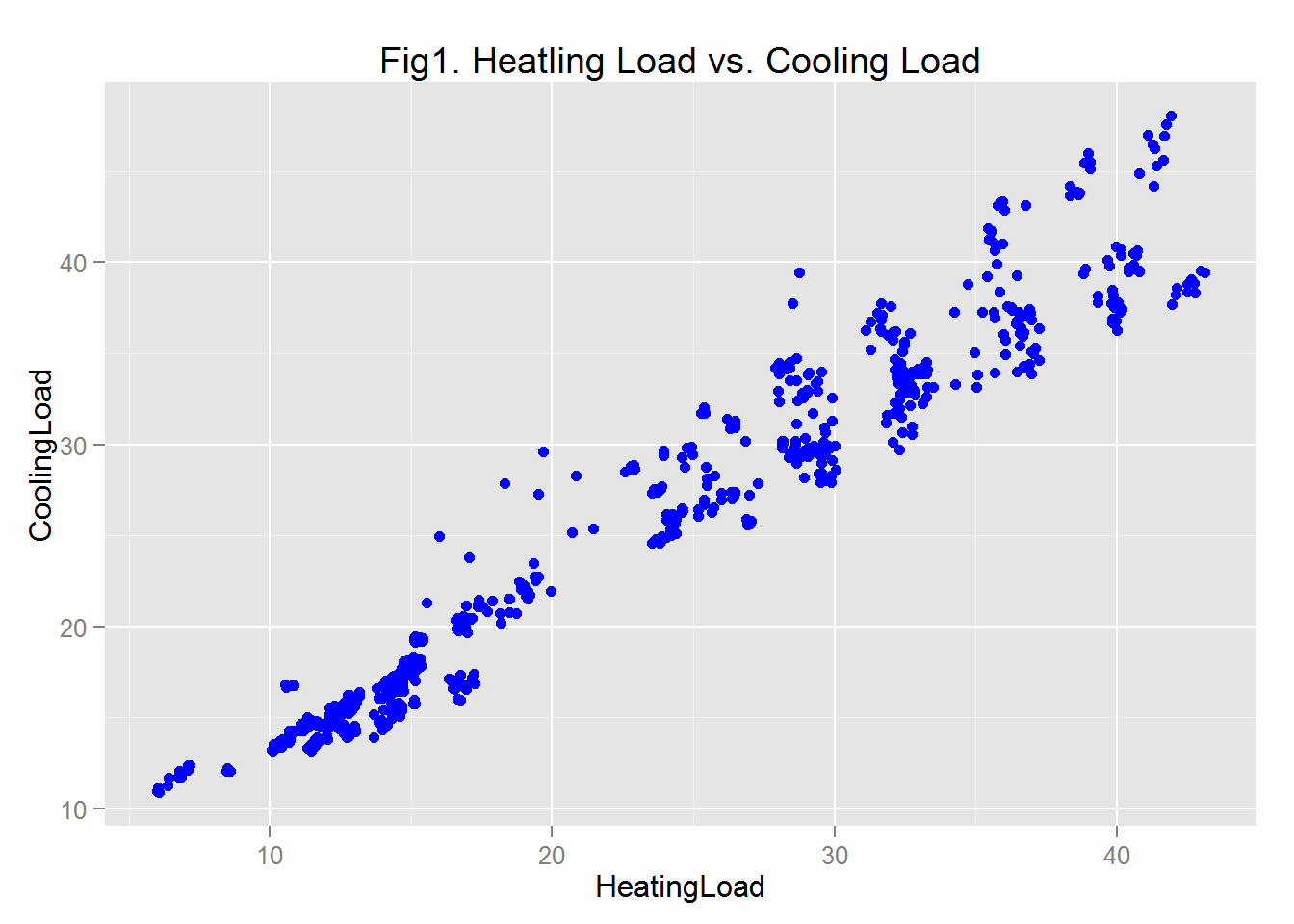The plot shows that the two response variables are highly correlated and predicting one of the variables will help to predict the other. Hebce, let’s remove the CoolingLoad variable

eeframe$CoolingLoad <- NULL Some of the variable are shown in the data as numeric variables, but actually they are categorical. Let’s covert these variables into categorical or factor variables. catList <- c("OverallHeight", "Orientation") eeframe[, catList] <- lapply(eeframe[, catList], function(x) as.factor(as.character(x))) Becauase of the difference in the values of the numeric vaiables, scaling and normalizing in important so that the variable with large values will not bias the training of the model. Here, the numeric vaiables are scaled. scaleList <- c("RelativeCompactness", "SurfaceArea", "WallArea", "RoofArea", "GlazingArea", "GlazingAreaDistribution") eeframe[, scaleList] <- lapply(eeframe[, scaleList], function(x) as.numeric(scale(x))) Let’s look at the summary of the data to check if the transformations are done as we expected. str(eeframe) ## 'data.frame': 768 obs. of 9 variables: ##$ RelativeCompactness    : num  2.04 2.04 2.04 2.04 1.28 ...
##  $SurfaceArea : num -1.78 -1.78 -1.78 -1.78 -1.23 ... ##$ WallArea               : num  -0.562 -0.562 -0.562 -0.562 0 ...
##  $RoofArea : num -1.47 -1.47 -1.47 -1.47 -1.2 ... ##$ OverallHeight          : Factor w/ 2 levels "3.5","7": 2 2 2 2 2 2 2 2 2 2 ...
##  $Orientation : Factor w/ 4 levels "2","3","4","5": 1 2 3 4 1 2 3 4 1 2 ... ##$ GlazingArea            : num  -1.76 -1.76 -1.76 -1.76 -1.76 ...
##  $GlazingAreaDistribution: num -1.81 -1.81 -1.81 -1.81 -1.81 ... ##$ HeatingLoad            : num  15.6 15.6 15.6 15.6 20.8 ...

Pair-wise scatter plots can help to visualization the relationship among variables. Let’s use the pair-wise scatter plots to analyze the relationships.

pairs(~ ., data = eeframe)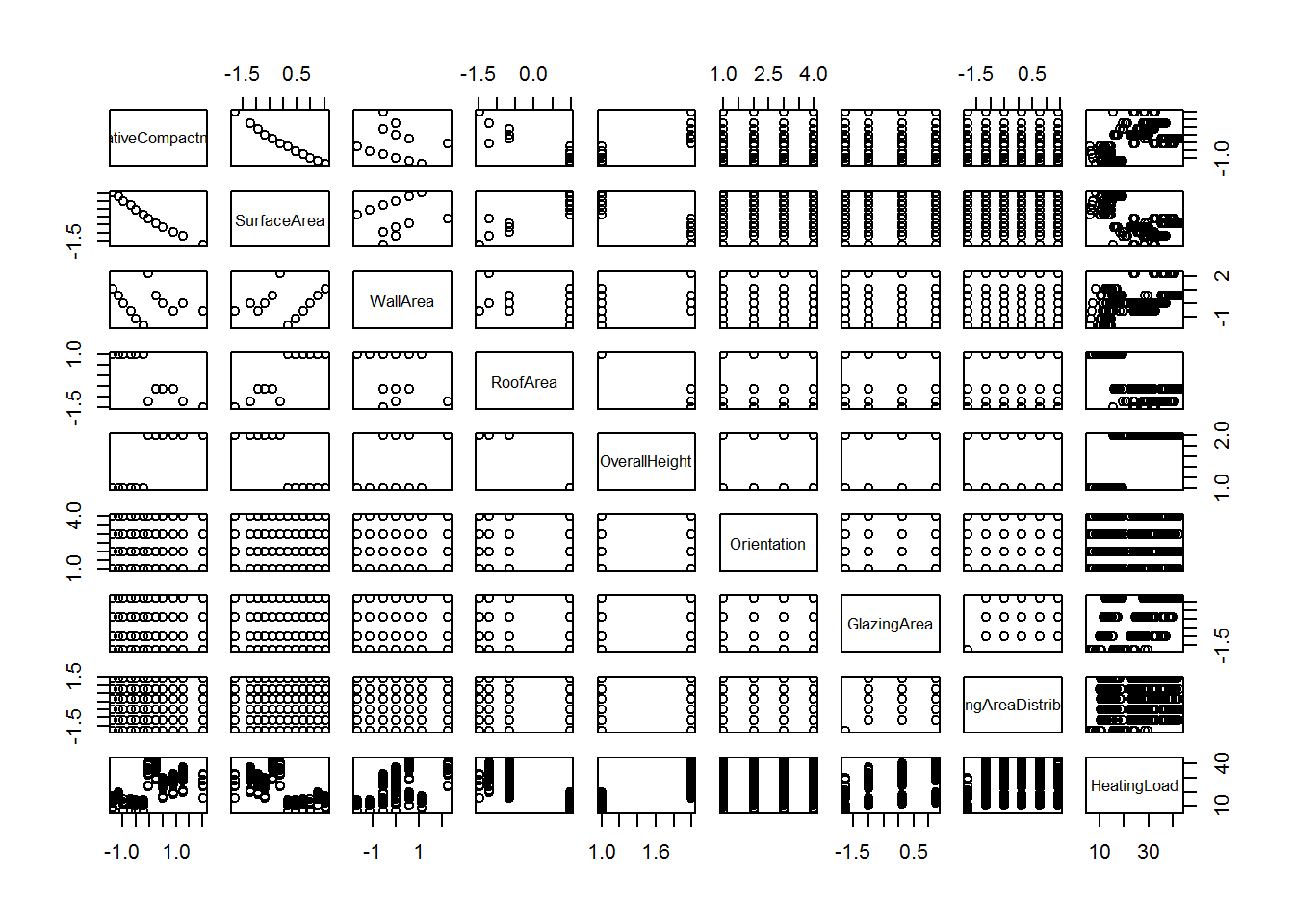The result of the scatter plot show that RelativeCompactness and SurfaceArea are highly correlated, and one of them can be removed. Moreover, HeatingLoad clusters into two groups for certain variables. For example, look at the cross plots between HeatingLoad and RelativeCompactness and SurfaceArea.

### Conditional Plots

plotCols <- c("RelativeCompactness",
"SurfaceArea")
plotEE <- function(x){
title <- paste("Figure2. Heating Load vs", x, "\n conditioned on OverallHeight and Orientation")
ggplot(eeframe, aes_string(x, "HeatingLoad")) +
geom_point(color="blue") +
facet_grid(OverallHeight ~ Orientation) +
ggtitle(title) +
stat_smooth(method = "lm", color='Brown')
}
lapply(plotCols, plotEE)
## []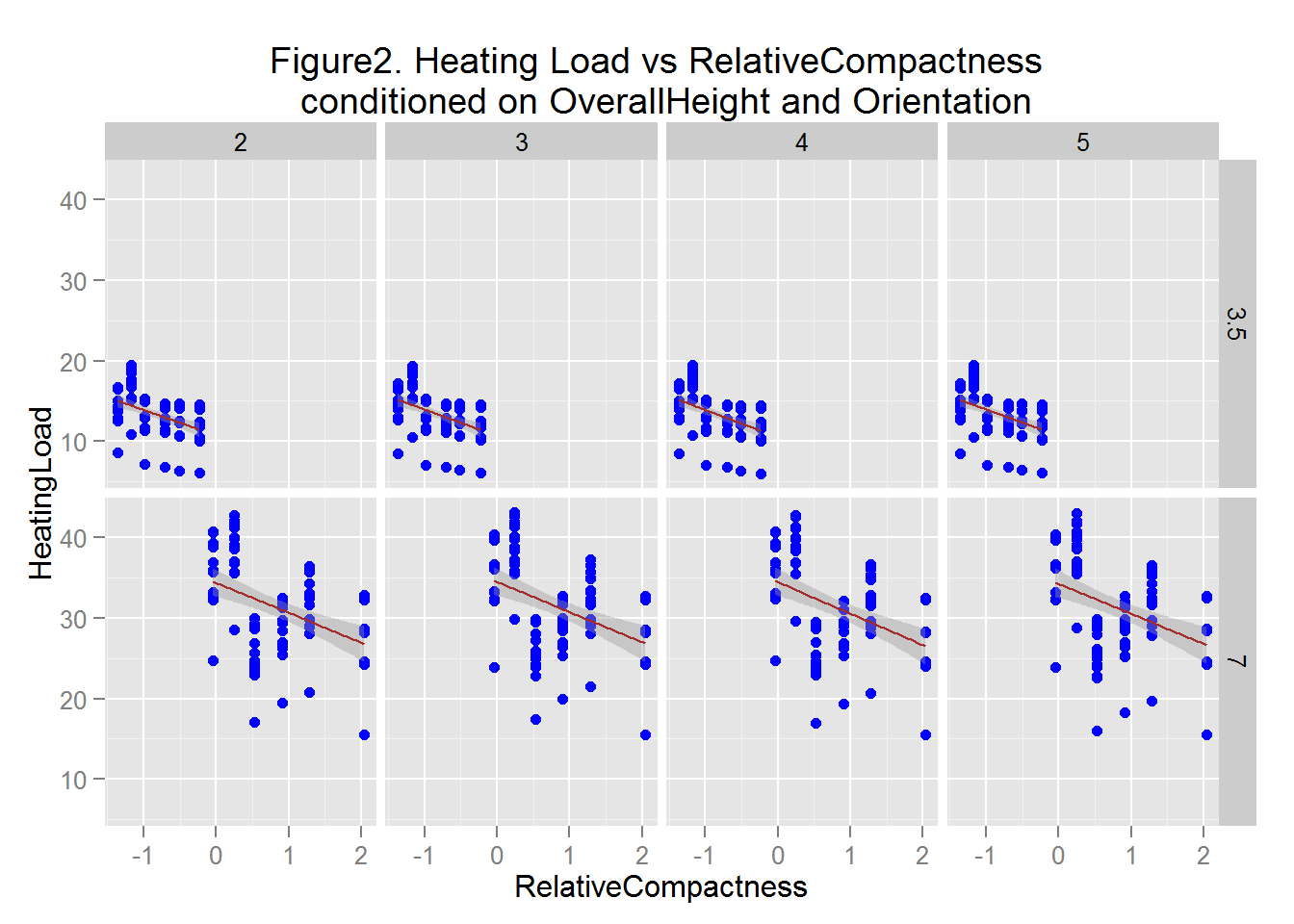##
## []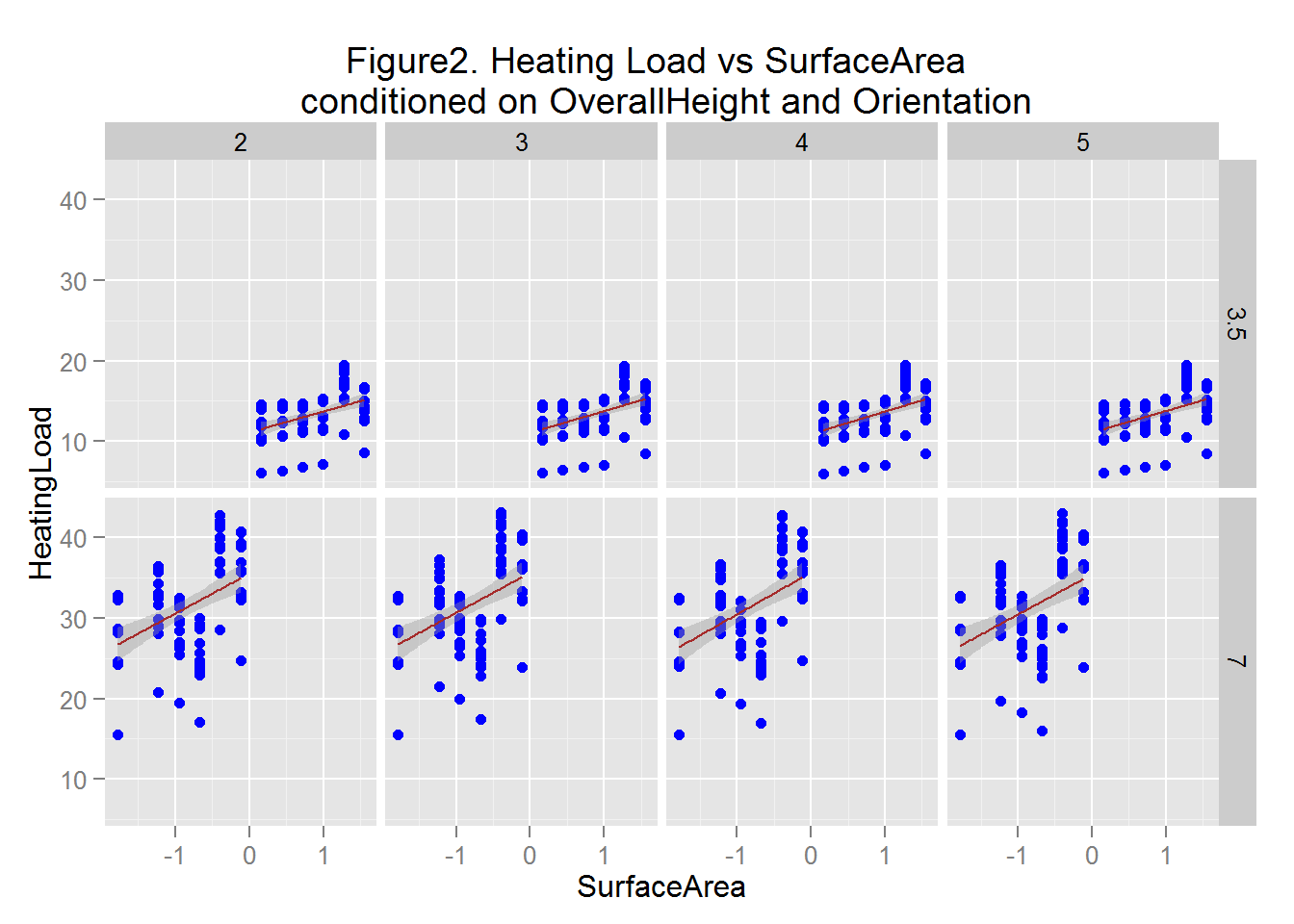The the conditional plots in Figure 2 shows that the range of values of HeatingLoad are quite different between the upper and lower rows; OverallHeight of 3.5 and 7, respectively. In fact, there is very little overlap in these values, indicating that OverallHeight is an important feature in these data. ** * The distribution of these data does not change significantly with the levels of Orientation, indicating it is not a significant feature. ??? There is a notable trend of HeatingLoad with respect to RelativeCompactness, indicating RelativeCompactness is a significant feature.

It is also noted that the relationship between the Heating Load and some of the predictor variables, namely Surface Area nad Relative Compacness is not linear. Transformation of the Surface Area may be needed here.

Histograms can also help us visualize the different levels of a variable. For example, let’s plot the two levels of the OverallHeight.

plotCols4 <- c("HeatingLoad")

eeHist <- function(x) {
title <- paste("Figure 3. Histogram of", x, "conditioned on OverallHeight")
ggplot(eeframe, aes_string(x)) +
geom_histogram( aes(y = ..density..,)) +
facet_grid(. ~ OverallHeight) +
ggtitle(title) +
geom_density(color="red")
}
lapply(plotCols4, eeHist)
## []
## stat_bin: binwidth defaulted to range/30. Use 'binwidth = x' to adjust this.
## stat_bin: binwidth defaulted to range/30. Use 'binwidth = x' to adjust this.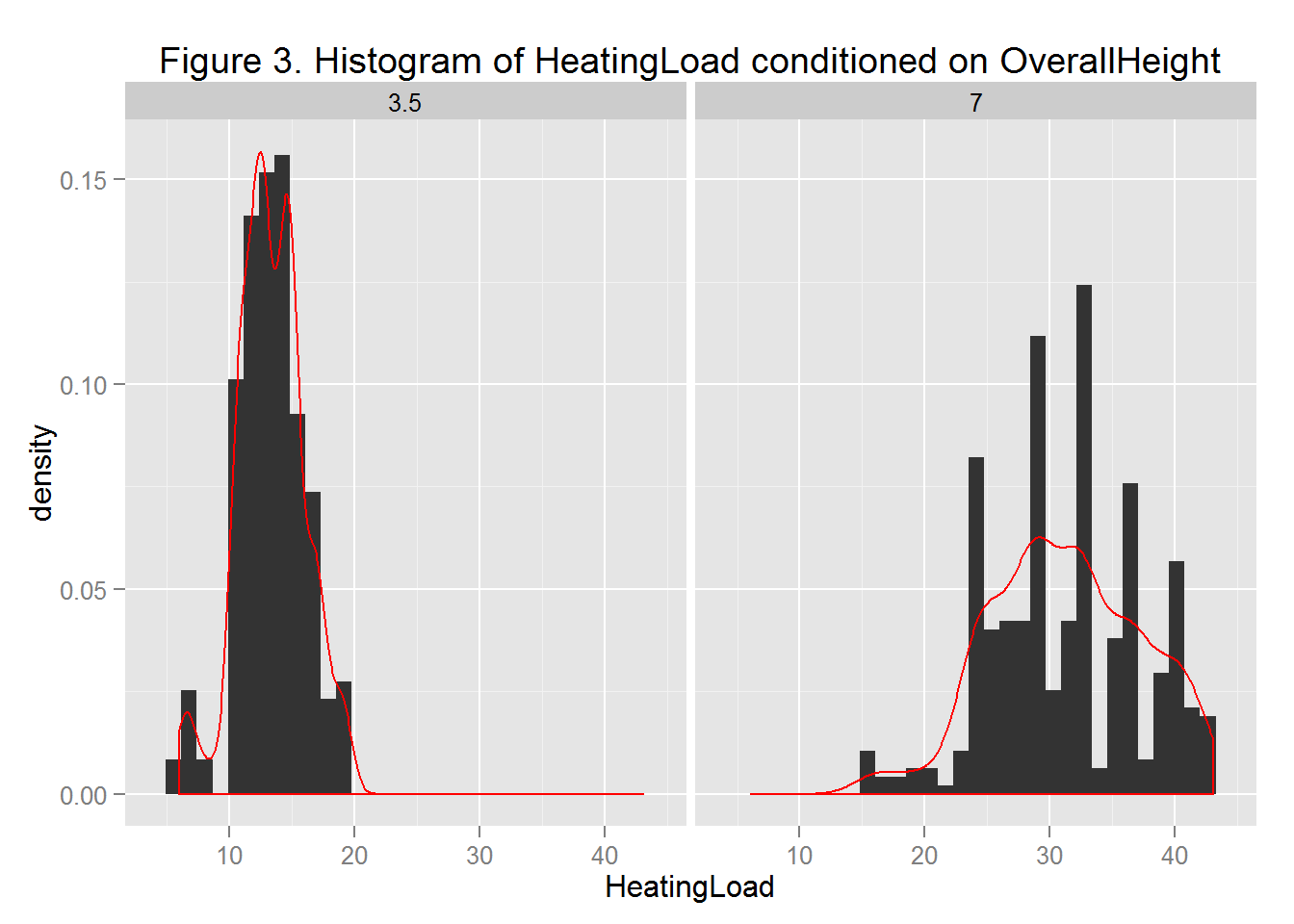The distributions of the Heating Load for the two values of the OverallHeight are different, with little overlap.

## Feature Engineering

It was noted from the conditional plots that some of the independent variables have nonlinear relationship with the dependent variable. Coverting these variables to polynomial variables may provide better fits. Let’s convert the Surface Area and the Relative Compactness to their squared and cube forms.

library(dplyr)
## Warning: package 'dplyr' was built under R version 3.2.2
##
## Attaching package: 'dplyr'
##
## The following objects are masked from 'package:stats':
##
##     filter, lag
##
## The following objects are masked from 'package:base':
##
##     intersect, setdiff, setequal, union
eeframe = mutate(eeframe,
RelativeCompactnessSqred = RelativeCompactness^2,
SurfaceAreaSqred = SurfaceArea^2,
RelativeCompactness3 = RelativeCompactness^3,
SurfaceArea3 = SurfaceArea^3)      

As shown below, the transfomation is done. Next, a linear regression model will be developed.

## 'data.frame':    768 obs. of  13 variables:
##  $RelativeCompactness : num 2.04 2.04 2.04 2.04 1.28 ... ##$ SurfaceArea             : num  -1.78 -1.78 -1.78 -1.78 -1.23 ...
##  $WallArea : num -0.562 -0.562 -0.562 -0.562 0 ... ##$ RoofArea                : num  -1.47 -1.47 -1.47 -1.47 -1.2 ...
##  $OverallHeight : Factor w/ 2 levels "3.5","7": 2 2 2 2 2 2 2 2 2 2 ... ##$ Orientation             : Factor w/ 4 levels "2","3","4","5": 1 2 3 4 1 2 3 4 1 2 ...
##  $GlazingArea : num -1.76 -1.76 -1.76 -1.76 -1.76 ... ##$ GlazingAreaDistribution : num  -1.81 -1.81 -1.81 -1.81 -1.81 ...
##  $HeatingLoad : num 15.6 15.6 15.6 15.6 20.8 ... ##$ RelativeCompactnessSqred: num  4.16 4.16 4.16 4.16 1.65 ...
##  $SurfaceAreaSqred : num 3.19 3.19 3.19 3.19 1.51 ... ##$ RelativeCompactness3    : num  8.5 8.5 8.5 8.5 2.12 ...
##  $SurfaceArea3 : num -5.68 -5.68 -5.68 -5.68 -1.85 ... ## Linear Regression Model Let’s divide the data into training and testing datasets. lmModel <- lm(HeatingLoad~., data=eeframe) summary(lmModel) ## ## Call: ## lm(formula = HeatingLoad ~ ., data = eeframe) ## ## Residuals: ## Min 1Q Median 3Q Max ## -7.7289 -1.2619 0.0731 1.4039 4.3714 ## ## Coefficients: (1 not defined because of singularities) ## Estimate Std. Error t value Pr(>|t|) ## (Intercept) 7.84443 0.65763 11.928 < 2e-16 *** ## RelativeCompactness 119.86752 4.74028 25.287 < 2e-16 *** ## SurfaceArea 122.43109 5.05524 24.219 < 2e-16 *** ## WallArea -5.79346 0.35086 -16.512 < 2e-16 *** ## RoofArea NA NA NA NA ## OverallHeight7 62.11010 1.82535 34.026 < 2e-16 *** ## Orientation3 0.06781 0.19714 0.344 0.731 ## Orientation4 -0.05297 0.19714 -0.269 0.788 ## Orientation5 -0.03750 0.19714 -0.190 0.849 ## GlazingArea 2.65544 0.07138 37.200 < 2e-16 *** ## GlazingAreaDistribution 0.31604 0.07138 4.427 1.09e-05 *** ## RelativeCompactnessSqred 83.08808 3.15964 26.297 < 2e-16 *** ## SurfaceAreaSqred -96.65523 3.35815 -28.782 < 2e-16 *** ## RelativeCompactness3 -2.06598 0.29682 -6.960 7.38e-12 *** ## SurfaceArea3 16.18554 0.67206 24.083 < 2e-16 *** ## --- ## Signif. codes: 0 '***' 0.001 '**' 0.01 '*' 0.05 '.' 0.1 ' ' 1 ## ## Residual standard error: 1.932 on 754 degrees of freedom ## Multiple R-squared: 0.964, Adjusted R-squared: 0.9634 ## F-statistic: 1552 on 13 and 754 DF, p-value: < 2.2e-16 Cofficient of determination value (R-squared) of 0.98 shows that the model is a good model. You can also see that Orientation and WallArea are not significant. Moreover, of the transformed variables, SurfaceArea3 and RelativeCompactnessSqred are more significant. Let’s remove the insignificant feature and rebuild the model. lmModel <- lm(HeatingLoad~.- RelativeCompactness - SurfaceArea - RoofArea - Orientation - SurfaceAreaSqred - GlazingAreaDistribution - RelativeCompactness3, data=eeframe) summary(lmModel) ## ## Call: ## lm(formula = HeatingLoad ~ . - RelativeCompactness - SurfaceArea - ## RoofArea - Orientation - SurfaceAreaSqred - GlazingAreaDistribution - ## RelativeCompactness3, data = eeframe) ## ## Residuals: ## Min 1Q Median 3Q Max ## -10.153 -1.347 0.033 1.310 7.462 ## ## Coefficients: ## Estimate Std. Error t value Pr(>|t|) ## (Intercept) 15.4799 0.2945 52.564 < 2e-16 *** ## WallArea 2.6764 0.1671 16.015 < 2e-16 *** ## OverallHeight7 15.5953 0.4091 38.123 < 2e-16 *** ## GlazingArea 2.7227 0.1049 25.945 < 2e-16 *** ## RelativeCompactnessSqred -1.0265 0.1253 -8.192 1.08e-15 *** ## SurfaceArea3 -0.4397 0.1124 -3.911 1e-04 *** ## --- ## Signif. codes: 0 '***' 0.001 '**' 0.01 '*' 0.05 '.' 0.1 ' ' 1 ## ## Residual standard error: 2.906 on 762 degrees of freedom ## Multiple R-squared: 0.9176, Adjusted R-squared: 0.917 ## F-statistic: 1697 on 5 and 762 DF, p-value: < 2.2e-16 The R-squared is now 0.97, but as you can see less number of features are used to get a reasonablly good model. eeframe$PredictedValues <- predict(lmModel, newdata=eeframe)

Now, let’s compute the residuals

eeframe$Resids <- eeframe$HeatingLoad - eeframe$PredictedValues ggplot(eeframe, aes(x = HeatingLoad, y = Resids , by = OverallHeight)) + geom_point(aes(color = OverallHeight)) + xlab("Heating Load") + ylab("Residuals") + ggtitle("Residuals vs Heating Load") + theme(text = element_text(size=20))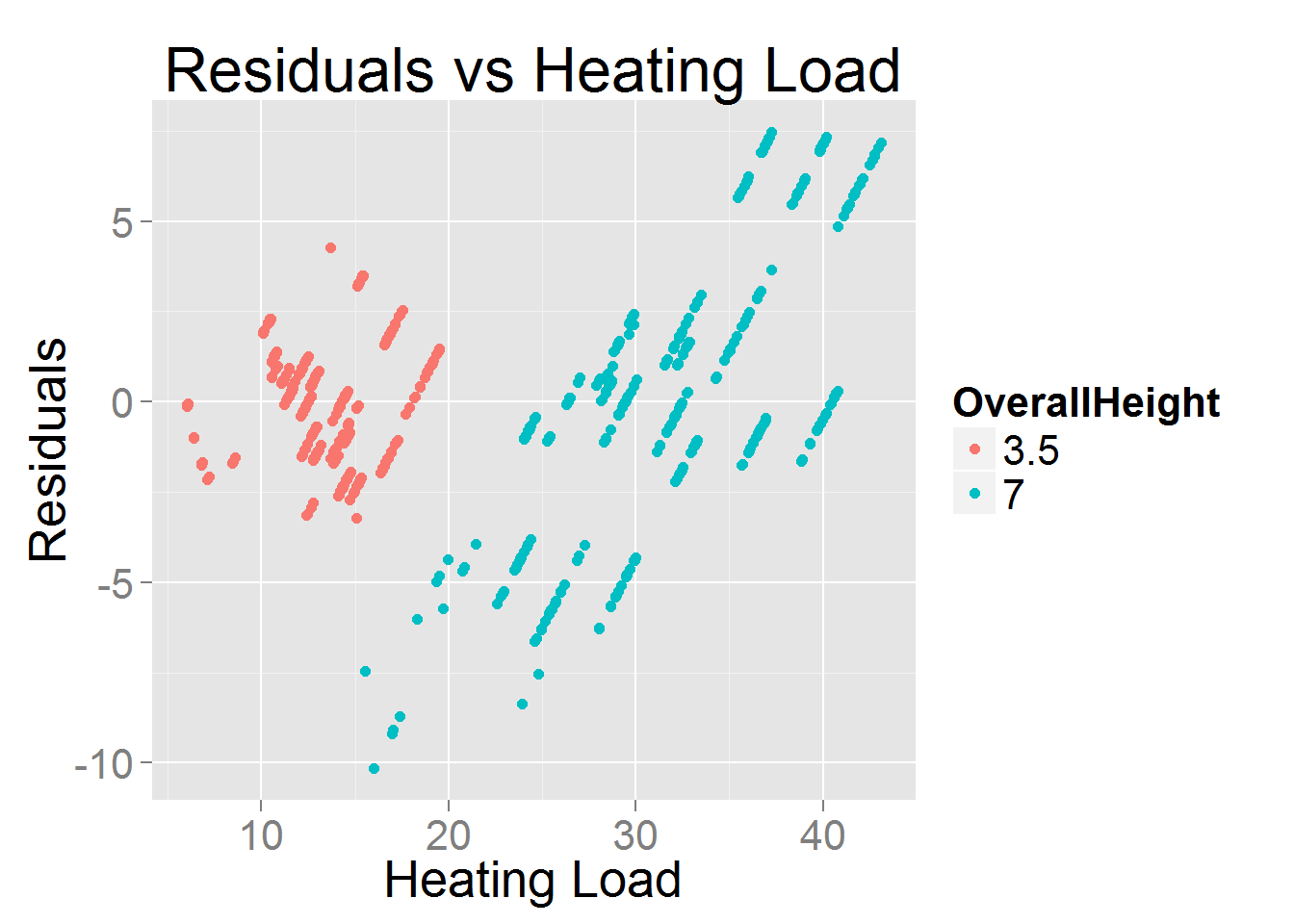The residual plot can show the quality of the model to some extent. In ideal case, the residuals should be randomly distributed. However, this not the case for our results. The seems to a trend specially for OverallHeight of 7. The Q-Q plots and the RMSE values attests this. ### Quantile-quantile normal plot of the residuals. Resids35 <- eeframe[eeframe$OverallHeight == 3.5, ]$Resid Resids7 <- eeframe[eeframe$OverallHeigh == 7, ]$Resid par(mfrow = c(1,2)) qqnorm(Resids35) qqnorm(Resids7)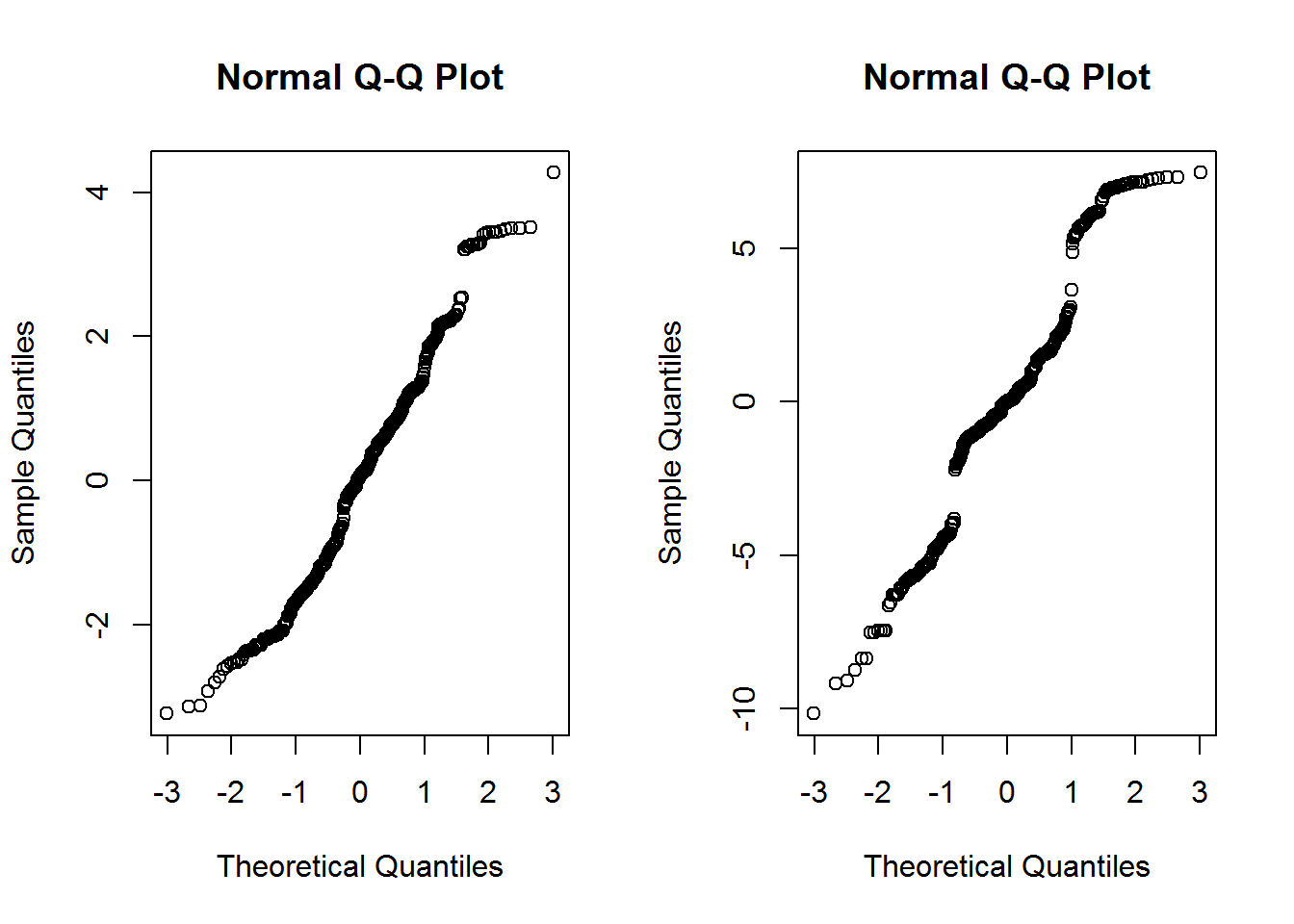par(mfrow = c(1,1)) Let’s compute the RMSE values of the residuals for both the overall and evaluation parts of the dataset. rmse <- function(x){ sqrt(sum(x^2)/length(x)) } residualFrame <- data.frame( rms_Overall = rmse(eeframe$Resids),
rms_35 = rmse(Resids35),
rms_7 = rmse(Resids7))

residualFrame
##   rms_Overall   rms_35    rms_7
## 1    2.894974 1.574389 3.779292